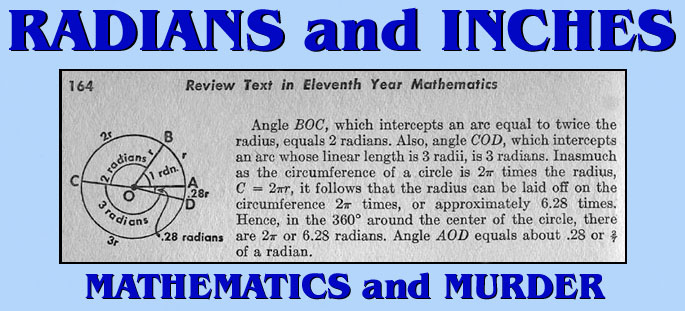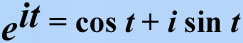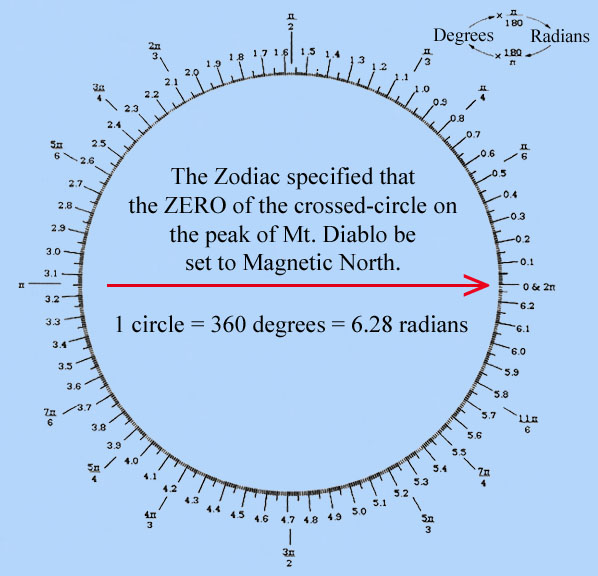[The excerpts below were written by David E. Joyce, Professor of Mathematics and Computer Science at Clark University.]

* EXCERPT: The concept of angle is one of the most important concepts in geometry. The concepts of equality, sums, and differences of angles are important and used throughout geometry, but the subject of trigonometry is based on the measurement of angles.

There are two commonly used units of measurement for angles. The more familiar unit of measurement is that of degrees. A circle is divided into 360 equal degrees, so that a right angle is 90°... Degrees may be further divided into minutes and seconds, but that division is not as universal as it used to be. Parts of a degree are now frequently referred to decimally. For instance seven and a half degrees is now usually written 7.5°. Each degree is divided into 60 equal parts called minutes. So seven and a half degrees can be called 7 degrees and 30 minutes, written 7° 30'. Each minute is further divided into 60 equal parts called seconds, and, for instance, 2 degrees 5 minutes 30 seconds is written 2° 5' 30". The division of degrees into minutes and seconds of angle is analogous to the division of hours into minutes and seconds of time.

Usually when a single angle is drawn on a xy-plane for analysis, we'll draw it with the vertex at the origin (0,0), one side of the angle along the x-axis, and the other side above the x-axis.The other common measurement for angles is radians. For this measurement, consider the unit circle (a circle of radius 1) whose center is the vertex of the angle in question. Then the angle cuts off an arc of the circle, and the length of that arc is the radian measure of the angle. It is easy to convert between degree measurement and radian measurement. The circumference of the entire circle is 2pi (pi is about 3.14159), so it follows that 360° equals 2pi radians. Hence, 1° equals pi/180 radians, and 1 radian equals 180/pi degrees.

Although the word "radian" was coined by Thomas Muir and/or James Thompson about 1870, mathematicians had been measuring angles that way for a long time. For instance, Leonhard Euler (1707-1783) in his Elements of Algebra explicitly said to measure angles by the length of the arc cut off in the unit circle. That was necessary to give his famous formula involving complex numbers that relates the sign and cosine functions to the exponential function where t is what was later called the radian measurment of the angle.